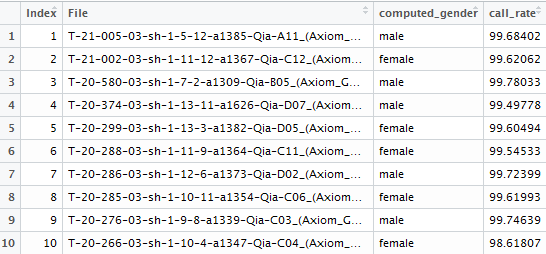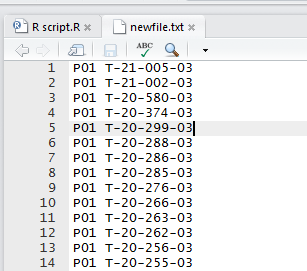# R语言：批量循环读取一系列excel文件install.packages(XLConnect)
library(XLConnect)

temp<-list.files(pattern="*.xls")     //生成一个新变量temp代表文件路径中所有后缀为xls的文件的文件名

a$plate=substr(as.character(temp),1,3) //生成变量plate，数值为temp里的plate名称（字符1到3） a$id=substr(a[,2],1,11)     //生成变量id, 值为第二列数据的前11个字符

ncol(a)   //看看a有多少个变量，新生成的plate和id变量为最后两个，假设为第58和59个变量

write.table(a[,c(58,59)],file = "newfile.txt",row.names=F, na="",col.names=FALSE, sep=" ",append = T,quote=F)

//生成txt文件newfile.txt为p01文件中的plate和id，展示如下for (i in 2: length(temp)){
b$row=substr(as.character(temp[i]),1,3) b$extract=substr(b[,2],1,11)
write.table(b[,c(58,59)],file = "newfile.txt",row.names=F, na="",col.names=FALSE, sep=" ",append = T,quote=F)
}

//命令翻译：对每一个i值，i从2到temp的最大值之间取值，生成一个文件newfile=之前P01的txt文件，下面四行是重复上面生成P01file的过程。

©️2019 CSDN 皮肤主题: 大白 设计师: CSDN官方博客